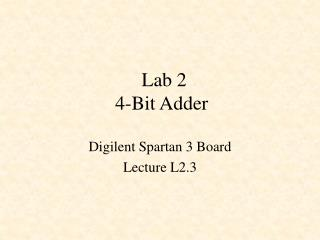DownloadDownload PresentationDownload Presentation- - - - - - - - - - - - - - - - - - - - - - - - - - - E N D - - - - - - - - - - - - - - - - - - - - - - - - - - -
##### Presentation Transcript

1. Lab 24-Bit Adder Digilent Spartan 3 Board Lecture L2.3

2. 4-Bit Adder component adder4 port( A : in std_logic_vector(3 downto 0); B : in std_logic_vector(3 downto 0); carry : out std_logic; S : out std_logic_vector(3 downto 0)); end component;

3. Top-level Design

4. library IEEE; use IEEE.STD_LOGIC_1164.all; use IEEE.std_logic_unsigned.all; entity Lab2 is port( mclk : in STD_LOGIC; SW : in STD_LOGIC_VECTOR(7 downto 0); BTN : in STD_LOGIC_VECTOR(3 downto 0); LD : out STD_LOGIC_VECTOR(7 downto 0); AtoG : out STD_LOGIC_VECTOR(6 downto 0); dp : out STD_LOGIC; AN : out STD_LOGIC_VECTOR(3 downto 0) ); end Lab2;

5. x7seg component x7seg port( x : in std_logic_vector(15 downto 0); cclk : in std_logic; clr : in std_logic; AtoG : out std_logic_vector(6 downto 0); AN : out std_logic_vector(3 downto 0)); end component;

6. Clock Divider signal cclk, carry: std_logic; signal clkdiv: std_logic_vector(23 downto 0); begin -- Divide the master clock (50Mhz) down to a lower frequency. process (mclk) begin if mclk = '1' and mclk'Event then clkdiv <= clkdiv + 1; end if; end process; cclk <= clkdiv(17); -- 381 Hz = 50000000 / 2^17

7. signal ground: std_logic_vector(2 downto 0); signal sum: std_logic_vector(3 downto 0); begin ground <= "000"; dp <= '1'; U1: adder4 port map (A => SW(7 downto 4), B => SW(3 downto 0), S => sum, carry => carry); U2: x7seg port map (x(15 downto 12) => SW(7 downto 4), x(11 downto 8) => SW(3 downto 0), x(7 downto 4) => sum, x(3 downto 1) => ground, x(0) => carry, clr => BTN(3), cclk => cclk, AN => AN, AtoG => AtoG); LD(7 downto 4) <= BTN; LD(3 downto 0) <= BTN;

8. signal ground: std_logic_vector(2 downto 0); signal sum: std_logic_vector(3 downto 0); begin ground <= "000"; dp <= '1'; U1: adder4 port map (A => SW(7 downto 4), B => SW(3 downto 0), S => sum, carry => carry); U2: x7seg port map (x(15 downto 12) => SW(7 downto 4), x(11 downto 8) => SW(3 downto 0), x(7 downto 4) => sum, x(3 downto 1) => ground, x(0) => carry, clr => BTN(3), cclk => cclk, AN => AN, AtoG => AtoG); LD(7 downto 4) <= BTN; LD(3 downto 0) <= BTN;

9. signal ground: std_logic_vector(2 downto 0); signal sum: std_logic_vector(3 downto 0); begin ground <= "000"; dp <= '1'; U1: adder4 port map (A => SW(7 downto 4), B => SW(3 downto 0), S => sum, carry => carry); U2: x7seg port map (x(15 downto 12) => SW(7 downto 4), x(11 downto 8) => SW(3 downto 0), x(7 downto 4) => sum, x(3 downto 1) => ground, x(0) => carry, clr => BTN(3), cclk => cclk, AN => AN, AtoG => AtoG); LD(7 downto 4) <= BTN; LD(3 downto 0) <= BTN;

10. signal ground: std_logic_vector(2 downto 0); signal sum: std_logic_vector(3 downto 0); begin ground <= "000"; dp <= '1'; U1: adder4 port map (A => SW(7 downto 4), B => SW(3 downto 0), S => sum, carry => carry); U2: x7seg port map (x(15 downto 12) => SW(7 downto 4), x(11 downto 8) => SW(3 downto 0), x(7 downto 4) => sum, x(3 downto 1) => ground, x(0) => carry, clr => BTN(3), cclk => cclk, AN => AN, AtoG => AtoG); LD(7 downto 4) <= BTN; LD(3 downto 0) <= BTN;